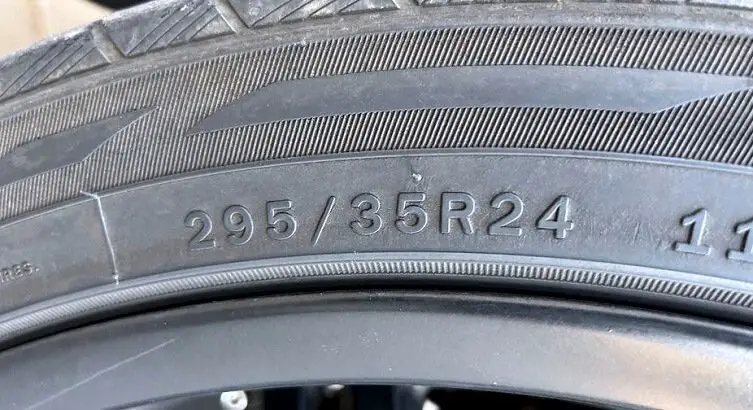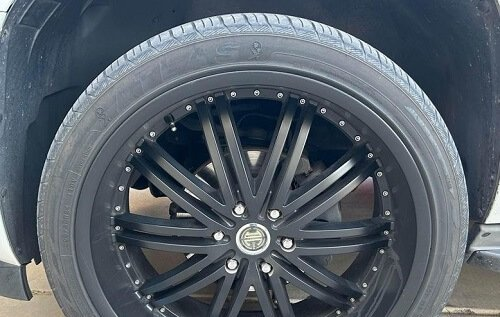# Tire Size 295 FAQWelcome to our “Tire Size 295 FAQ” guide, where we answer some of the most frequently asked questions about 295-width tires. As tire sizing and selection play a crucial role in your vehicle’s performance, safety, and overall driving experience, it’s essential to understand the ins and outs of this specific tire size.

### What Size Tires Are 295?

The tire size “295” typically refers to the tire’s width in millimeters. It is usually accompanied by two other numbers that provide additional information about the tire’s size: the aspect ratio and the rim diameter.

So, for example, a tire size of “295/35R20” indicates that the tire is 295 millimeters wide, has an aspect ratio of 35 (meaning the height of the tire is 35% of its width), and fits on a 20-inch rim.

## Are 295 Tires The Same As 33?

The 295 tires are not exactly the same as 33-inch tires, but they are quite close in size. “295” represents the tread width in millimeters, while “33” denotes the tire’s diameter in inches. To compare the overall diameter of a 295 tire to a 33-inch tire, we can use the same calculation method outlined previously, assuming a similar aspect ratio and rim diameter.

Let’s use a 295/70/17 tire as an example:

1. Measure the exact sidewall height: Multiply the tread width (295 mm) by the sidewall height (70%) to get 206.5 mm. Since a tire has two sidewalls, multiply 206.5 mm by 2 for a total sidewall height of 413 mm.
2. Convert the rim diameter to metric: Convert the 17-inch rim diameter to millimeters by multiplying it by 25.4, resulting in 431.8 millimeters.
3. Calculate the overall tire diameter: Add the total sidewall height (413 mm) and the rim diameter (431.8 mm) to get 844.8 mm.

To find the tire diameter in inches, divide the total diameter (844.8 mm) by 25.4. This results in approximately 33.3 inches, which is close to 33 inches but slightly larger.

In summary, 295 tires are not exactly the same as 33-inch tires but are quite similar in size. The overall diameter of a 295/70/17 tire is around 33.3 inches, making it slightly larger than a 33-inch tire.### How Tall Is A 295 Tire?

The overall diameter or height of a 295 tire depends on the aspect ratio (sidewall height) and the rim diameter. To calculate the height, we need the full tire size, which includes the aspect ratio and rim diameter, expressed as 295/XX/YY, where XX is the aspect ratio and YY is the rim diameter in inches.

For example, let’s calculate the height of a 295/70/17 tire:

1. Measure the exact sidewall height: Multiply the tread width (295 mm) by the sidewall height (70%) to get 206.5 mm. Since a tire has two sidewalls, multiply 206.5 mm by 2 for a total sidewall height of 413 mm.
2. Convert the rim diameter to metric: Convert the 17-inch rim diameter to millimeters by multiplying it by 25.4, resulting in 431.8 millimeters.
3. Calculate the overall tire diameter: Add the total sidewall height (413 mm) and the rim diameter (431.8 mm) to get 844.8 mm.

To find the tire diameter in inches, divide the total diameter (844.8 mm) by 25.4. This results in approximately 33.3 inches.

### How Wide Are 295 Tires?

The number “295” in a tire size represents the tire’s tread width in millimeters. Therefore, a 295 tire is 295 millimeters wide. To convert this width to inches, divide by 25.4 (since there are 25.4 millimeters in an inch):

• 295 mm / 25.4 = 11.61 inches

So, a 295 tire is approximately 11.61 inches wide.

### How Much Wider Is A 295 Tire Than A 275?

Assuming you’re referring to the width of a tire in millimeters, a 295 tire is 20 millimeters wider than a 275 tire.

Specifically, the numbers 275 and 295 refer to the tire’s width in millimeters, measured from sidewall to sidewall. So a 275 tire has a width of 275 millimeters, while a 295 tire has a width of 295 millimeters.

Therefore, the difference in width between a 295 tire and a 275 tire is 295 – 275 = 20 millimeters. In inches, this would be a difference of about 0.79 inches (since 1 inch = 25.4 millimeters).

### 295 Tire Cost

Tire prices can vary significantly based on the brand, model, size, type of tire, location, and time of purchase. To give you a general idea of the cost for 295-width tires, you can expect to pay anywhere from \$150 to \$400 per tire. High-performance or premium brands may cost more, while budget options may be cheaper.

Keep in mind that these are approximate figures and can change depending on various factors. To get accurate pricing information for your specific requirements, it is recommended to check with local tire retailers or online stores for the latest prices and availability.

Scroll to Top